# MA.912.F.2.3Export Print
Given the graph or table of f(x) and the graph or table of f(x)+k,kf(x), f(kx) and f(x+k), state the type of transformation and find the value of the real number k.

### Clarifications

Clarification 1: Within the Algebra 1 course, functions are limited to linear, quadratic and absolute value.
General Information
Subject Area: Mathematics (B.E.S.T.)
Strand: Functions
Status: State Board Approved

## Benchmark Instructional Guide

### Terms from the K-12 Glossary

• Transformation
• Translation

### Vertical Alignment

Previous Benchmarks

Next Benchmarks

### Purpose and Instructional Strategies

In grade 8, students performed single transformations on two-dimensional figures. In Algebra I, students identified the effects of single transformations on linear, quadratic and absolute value functions. In Geometry, students performed multiple transformations on two-dimensional figures. In Mathematics for College Algebra, students determine the type of transformations on linear, quadratic, exponential, logarithmic and absolute value functions.
• Instruction includes identifying function transformations involving a combination of translations, dilations and reflections, and determining the value of the real number that defines each of the transformations.
• Transformations can be either horizontal (changes to the input: $x$) or vertical (changes to the output: $f$($x$)).
• There are three different types of transformations: translations, dilations, and reflections.
• By combining single transformations, a parent function can become a more advanced function.
$f$($x$) = $x$ → $h$($x$) = $A$$f$($B$($x$ + $C$) + $D$
Example: $f$($x$) = $x$2 $g$($x$) = 2($x$ + 3)2 + 5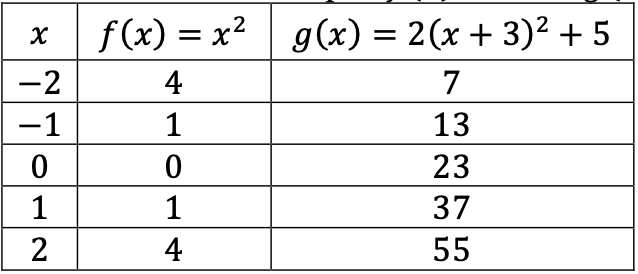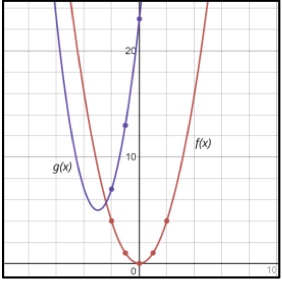• Using a graphing utility can help students understand how changing the value of the real numbers in the function’s equation change its graph.
• Encourage student’s discussion about the effects of changing the value of the real numbers $A$, $B$, $C$ and $D$ in the equation of the function. Ask them to generalize their findings.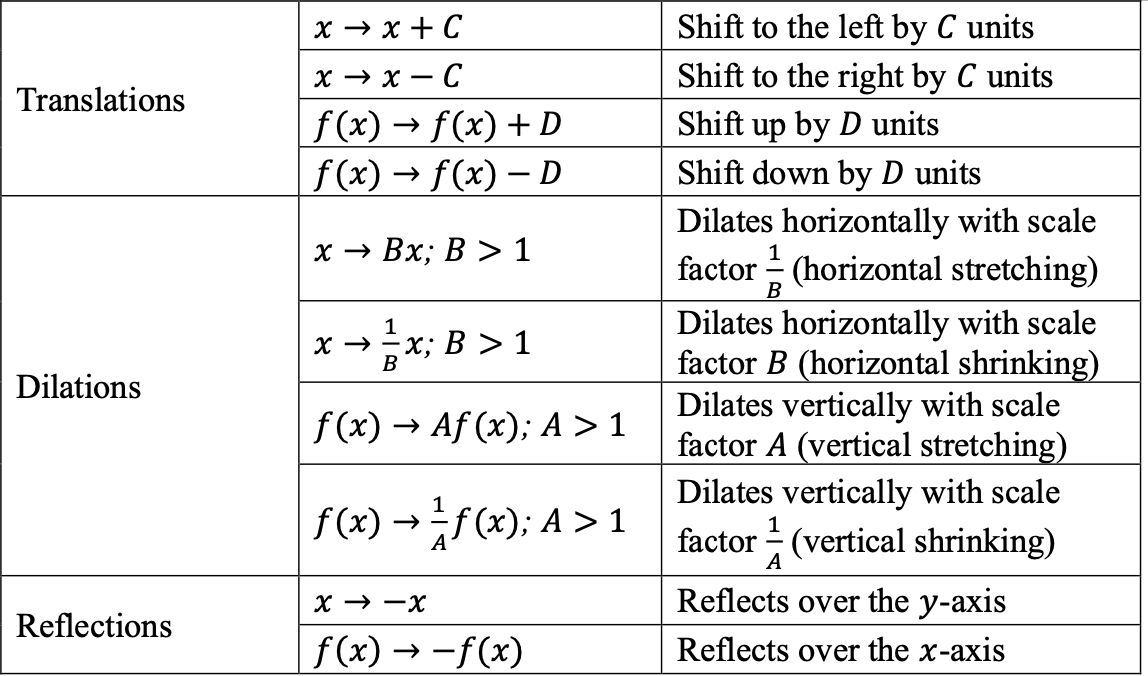### Common Misconceptions or Errors

• Some students may have difficulty seeing the impact of a transformation when comparing tables and graphs. In these cases, encourage students to convert the graph to a second table, using the same domain as the first table. This should aid in comparisons.
• Similar to writing functions in vertex form, students may confuse effect of the sign of $k$ in $f$($x$ + $k$). Direct these students to examine a graph of the two functions to see that the horizontal shift is opposite of the sign of $k$
• Vertical stretch/compression can be hard for students to see on linear functions initially and they may interpret stretch/compression as rotation. Introduce the effects of $k$$f$($x$) and $f$($k$$x$) by using a quadratic or absolute value function first before analyzing the effect on a linear function.
• Students may think that a vertical and horizontal stretch from $k$$f$($x$) and $f$($k$$x$) look the same. For linear and quadratic functions, it can help to have a non-zero $y$-intercept to visualize the difference.

• A graph and table, which represents an absolute value function, are shown below. Describe and determine the value of the real number that defines the transformation from $f$($x$) to $g$($x$).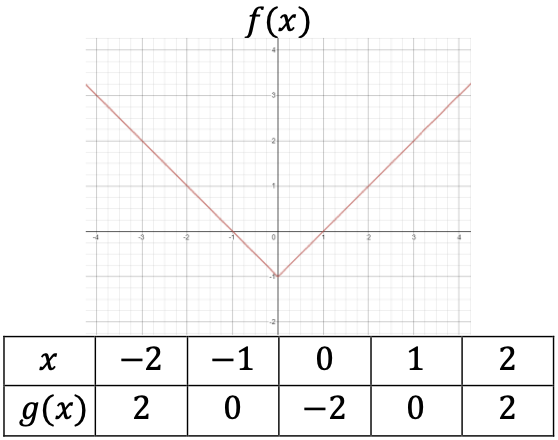• Describe the transformations that maps the function $f$($x$) = 2$x$ to each of the following.
$f$($x$) = 2$x$-2  $g$($x$) = 4$x$+ 3
$h$($x$) = 2$x$ − 3 $b$($x$)= 3(2$x$ + 1)+2

### Instructional Items

Instructional Item 1
• Considering the graph of $f$($x$) and $g$($x$) below, describe the transformation and determine the value of the real number, $k$, that defines the transformation from $f$($x$) to $g$($x$).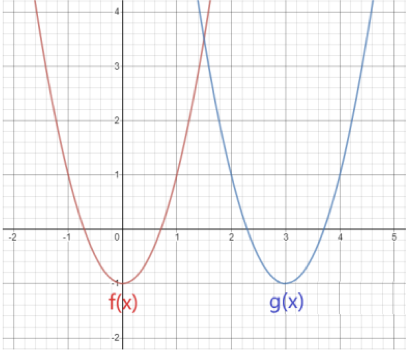Instructional Item 2
• Considering the table below, describe the transformation and determine the value of the real number, $k$, that defines the transformation from $f$($x$) to $g$($x$).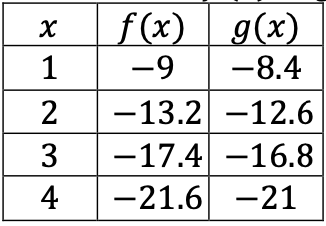*The strategies, tasks and items included in the B1G-M are examples and should not be considered comprehensive.

## Related Courses

This benchmark is part of these courses.
1200320: Algebra 1 Honors (Specifically in versions: 2014 - 2015, 2015 - 2022, 2022 and beyond (current))
1200330: Algebra 2 (Specifically in versions: 2014 - 2015, 2015 - 2022, 2022 and beyond (current))
1200340: Algebra 2 Honors (Specifically in versions: 2014 - 2015, 2015 - 2022, 2022 and beyond (current))
1200400: Foundational Skills in Mathematics 9-12 (Specifically in versions: 2014 - 2015, 2015 - 2022, 2022 and beyond (current))
7912095: Access Algebra 2 (Specifically in versions: 2016 - 2018, 2018 - 2019, 2019 - 2022, 2022 and beyond (current))
1200710: Mathematics for College Algebra (Specifically in versions: 2022 and beyond (current))
1209315: Mathematics for ACT and SAT (Specifically in versions: 2022 and beyond (current))

## Related Access Points

Alternate version of this benchmark for students with significant cognitive disabilities.
MA.912.F.2.AP.3:

Given the graph of a given function after replacing f(x) by f(x) + k and f(x + k), kf(c), for specific values of k select the type of transformation and find the value of the real number k.

## Related Resources

Vetted resources educators can use to teach the concepts and skills in this benchmark.

## Formative Assessment

Write the Equations:

Students are given the graphs of three absolute values functions and are asked to write the equation of each.

Type: Formative Assessment

## Lesson Plans

This lesson introduces students to the quadratic parent function, as well as reinforces some key features of quadratic functions. It allows students to explore basic transformations of quadratic functions and provides a note-taking sheet for students to organize their learning. There is a "FUN" cut and paste activity for students to match quadratic graphs with verbal descriptions and their equations.

Type: Lesson Plan

This lesson covers quadratic translations as they relate to vertex form of a quadratic equation. Students will predict what will happen to the graph of a quadratic function when more than one constant is in a quadratic equation. Then, the students will graph quadratic equations in vertex form using their knowledge of the translations of a quadratic function, as well as describe the translations that occur. Students will also identify the parent function of any quadratic function as $f\left(x\right)={x}^{2}$.

Type: Lesson Plan

In this lesson, students will investigate the changes to the graph of a quadratic function when the function is modified in four different ways by inclusion of an additive or multiplicative constant. Students will work in groups to graph quadratic functions, prepare a display of their functions, and determine how the modification affects the graph of the quadratic function. Then, students participate in a gallery walk, where members of each group will share their findings with a small group of students. At the end, there is a class discussion to see if everyone had similar findings and to solidify the knowledge of translating quadratic functions.

Type: Lesson Plan

## Original Student Tutorial

Dilations...The Effect of k on a Graph:

Visualize the effect of using a value of k in both kf(x) or f(kx) when k is greater than zero in this interactive tutorial.

Type: Original Student Tutorial

## MFAS Formative Assessments

Write the Equations:

Students are given the graphs of three absolute values functions and are asked to write the equation of each.

## Original Student Tutorials Mathematics - Grades 9-12

Dilations...The Effect of k on a Graph:

Visualize the effect of using a value of k in both kf(x) or f(kx) when k is greater than zero in this interactive tutorial.

## Student Resources

Vetted resources students can use to learn the concepts and skills in this benchmark.

## Original Student Tutorial

Dilations...The Effect of k on a Graph:

Visualize the effect of using a value of k in both kf(x) or f(kx) when k is greater than zero in this interactive tutorial.

Type: Original Student Tutorial

## Parent Resources

Vetted resources caregivers can use to help students learn the concepts and skills in this benchmark.